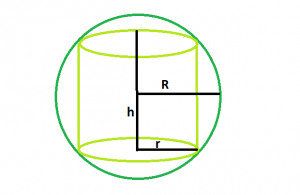GeeksforGeeks App
Open AppBrowser
Continue

# Volume of largest right circular cylinder within a Sphere

Given a sphere of radius. The task is to find volume of the biggest right circular cylinder that can be inscribed within it.
Examples

```Input : R = 4
Output : 77.3495

Input : R = 5
Output : 151.073```Approach
let r be the radius of the right circular cylinder, and h be it’s height.
Volume of the cylinder, V = π*r2*h
Also, r2 = R2 – h2
or, V = π*(R2 – h2)*h
or, dV/dh = π*(R2 – 3*h2)
Setting it to zero, we get h = R/√3
So, Vmax = 2πR3/3√3
Below is the implementation of the above approach:

## C++

 `// C++ Program to find the biggest right circular cylinder``// that can be fit within a sphere` `#include ``using` `namespace` `std;` `// Function to find the biggest right circular cylinder``float` `cyl(``float` `R)``{` `    ``// radius cannot be negative``    ``if` `(R < 0)``        ``return` `-1;` `    ``// volume of cylinder``    ``float` `V = (2 * 3.14 * ``pow``(R, 3)) / (3 * ``sqrt``(3));``    ``return` `V;``}` `// Driver code``int` `main()``{``    ``float` `R = 4;` `    ``cout << cyl(R) << endl;` `    ``return` `0;``}`

## Java

 `// Java Program to find the biggest``// right circular cylinder that can``// be fit within a sphere``import` `java.io.*;` `class` `GFG``{` `// Function to find the biggest``// right circular cylinder``static` `float` `cyl(``float` `R)``{` `    ``// radius cannot be negative``    ``if` `(R < ``0``)``        ``return` `-``1``;` `    ``// volume of cylinder``    ``float` `V = (``float``)((``2` `* ``3.14` `* Math.pow(R, ``3``)) /``                      ``(``3` `* Math.sqrt(``3``)));``    ``return` `V;``}` `// Driver code``public` `static` `void` `main (String[] args)``{``    ``float` `R = ``4``;` `    ``System.out.print( cyl(R));``}``}` `// This code is contributed by anuj_67..`

## Python 3

 `# Python 3 Program to find the biggest``# right circular cylinder that can be``# fit within a sphere``import` `math` `# Function to find the biggest right``# circular cylinder``def` `cyl(R):``    ` `    ``# radius cannot be negative``    ``if` `(R < ``0``):``        ``return` `-``1` `    ``# volume of cylinder``    ``V ``=` `((``2` `*` `3.14` `*` `math.``pow``(R, ``3``)) ``/``                ``(``3` `*` `math.sqrt(``3``)));``    ``return` `float``(V)` `# Driver code``R ``=` `4``print``(cyl(R))` `# This code is contributed``# by PrinciRaj1992`

## C#

 `// C# Program to find the biggest``// right circular cylinder that can``// be fit within a sphere``using` `System;` `class` `GFG``{` `// Function to find the biggest``// right circular cylinder``static` `float` `cyl(``float` `R)``{` `    ``// radius cannot be negative``    ``if` `(R < 0)``        ``return` `-1;` `    ``// volume of cylinder``    ``float` `V = (``float``)((2 * 3.14 * Math.Pow(R, 3)) /``                             ``(3 * Math.Sqrt(3)));``    ``return` `V;``}` `// Driver code``public` `static` `void` `Main ()``{``    ``float` `R = 4;` `    ``Console.WriteLine( cyl(R));``}``}` `// This code is contributed by shs`

## PHP

 ``

## Javascript

 ``

Output:

`77.3495`

Time Complexity: O(1)

Auxiliary Space: O(1)

My Personal Notes arrow_drop_up